Home | | Maths 6th Std | Construction of Perpendicular Lines

# Construction of Perpendicular Lines

In Geometry, to measure the height of figures, we use perpendicular lines. Using a set square, find the height of the given figures.

Construction of Perpendicular Lines

1. Introduction

Have you ever noticed that the wall and floor are always perpendicular to each other? So, to measure our heights, we make use of scale represented on the walls as shown in the figure.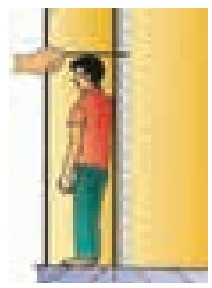In Geometry, to measure the height of figures, we use perpendicular lines. Using a set square, find the height of the given figures.Let us learn to construct perpendicular lines by using set square.

2. Set Squares

The set squares are two triangle shaped instruments in the Geometry Box. Each of them has a right angle. One set square has the angles 30°, 60°, 90° and the other set square has the angles 45°, 45°, 90°. The perpendicular edges are graduated in centimetres.

Set squares have several uses:

• To construct the specific angles 30° , 45° , 60° , 90°

• To draw parallel and perpendicular lines

• To measure the height of the shapes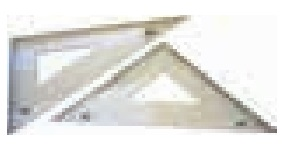If the perpendicular from P meets AB at Q, the point Q is called the foot of the perpendicular from P to AB and the symbol " " means “is perpendicular to”. i.e., PQ AB

Example 5:

Construct a line perpendicular to the given line at a point on the line.

Step 1: Draw a line AB and take a point P anywhere on the line.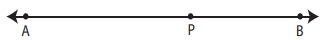Step 2: Place the set square on the line in such a way that the vertex which forms right angle coincides with P and one arm of the right angle coincides with the line AB.Step 3: Draw a line PQ through P along the other arm of the right angle of the set square.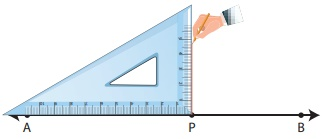Step 4: The line PQ is perpendicular to the line AB at P. That is, PQ AB and APQ = BPQ = 90° .Example 6:

Construct a line perpendicular to the given line through a point above it.

Step 1: Draw a line PQ. Take a point X anywhere above the line PQ.Step 2: Place one of the arms of the right angle of a set square along the line PQ and the other arm of its right angle touches the point X.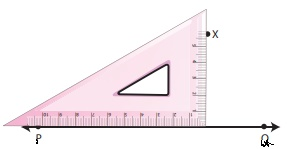Step 3: Draw a line through the point meeting PQ at Y.Step 4: The line XY is perpendicular to the line PQ at Y. That is, XY PQTags : Geometry | Term 2 Chapter 4 | 6th Maths , 6th Maths : Term 2 Unit 4 : Geometry
Study Material, Lecturing Notes, Assignment, Reference, Wiki description explanation, brief detail
6th Maths : Term 2 Unit 4 : Geometry : Construction of Perpendicular Lines | Geometry | Term 2 Chapter 4 | 6th Maths